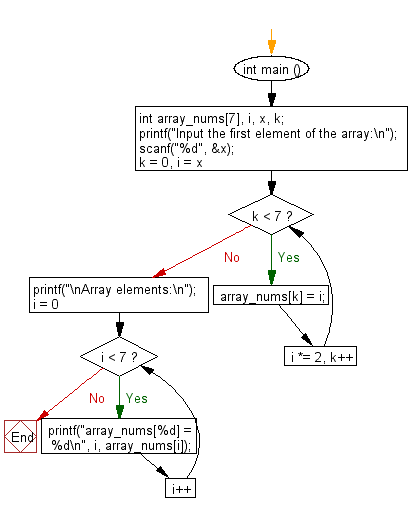﻿ C : Fill the array with the double value of the previous

# C Exercises: Array fill, replace each subsequent position of the array by the double value of the previous

## C Basic Declarations and Expressions: Exercise-125 with Solution

Write a C program that reads an array of integers (length 7), and replaces the first element of the array by a given number and replaces each subsequent position of the array by the double value of the previous.

Sample Solution:

C Code:

``````#include <stdio.h>

int main () {
int array_nums, i, x, k;

// Prompt user for the first element of the array
printf("Input the first element of the array:\n");
scanf("%d", &x);

// Loop to generate array elements by doubling 'x' in each iteration
for (k = 0, i = x; k < 7; i *= 2, k++) {
array_nums[k] = i; // Assign the calculated value to the array
}

// Print a message indicating the array elements will be displayed
printf("\nArray elements:\n");

// Loop to print each element of the array
for (i = 0; i < 7; i++) {
printf("array_nums[%d] = %d\n", i, array_nums[i]);
}

return 0; // End of program
}
``````

Sample Output:

```Input the first element of the array:
5

Array elements:
array_nums = 5
array_nums = 10
array_nums = 20
array_nums = 40
array_nums = 80
array_nums = 160
array_nums = 320
```

Flowchart:C programming Code Editor:

What is the difficulty level of this exercise?

Test your Programming skills with w3resource's quiz.

﻿